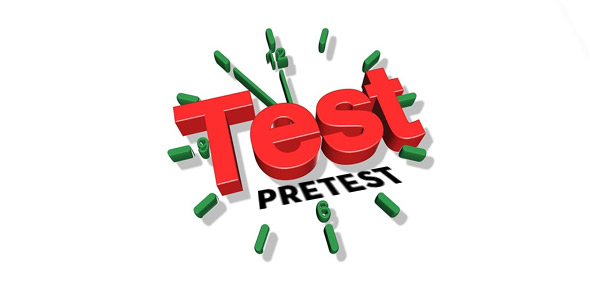# Atoms, Compounds, And Elements Pretest

10 Questions | Total Attempts: 305SettingsThis quiz is a pretest to the physical science unit (8th grade level) which covers atomic theory, atomic structure, molecules, compounds, and elements.

• 1.
What contribution to the atomic theory did Neils Bohr make?
• A.

He discovered the electron.

• B.

He theorized a model of atomic structure

• C.

He discovered the proton.

• D.

He viewed an atom for the first time using an instrument.

• 2.
Which of the following are parts of an atom? (Choose all that apply)
• A.

Neutron

• B.

Compound

• C.

Electron

• D.

Nucleus

• E.

Atomic bond

• F.

Proton

• G.

Element

• H.

Electron shell

• 3.
Where in the atom are the electrons found?
• A.

Inside the nucleus

• B.

Outside the nucleus

• C.

Orbitting through the nucleus

• 4.
What makes an atom able to form a compound?
• A.

Same number of protons as electrons

• B.

Different number of neutrons than protons

• C.

Unfilled outer electron shells

• D.

A positive or negative charge of the nucleus

• 5.
What charge does the nucleus of the atom have?
• A.

Positive

• B.

Neutral

• C.

Negative

• 6.
Which scientists was the first to use the term "atom"?
• A.

Democritus

• B.

John Dalton

• C.

JJ Thomson

• D.

Albert Einstein

• 7.
How does an isotope differ from another atom of the same element?
• A.

The number of neutrons is different

• B.

The number of protons is different

• C.

The number of electrons is different

• D.

The shape is different

• 8.
A pure substance that cannot be separated into simpler substances is called a(n)
• A.

Isotope

• B.

Atom

• C.

Element

• D.

Compound

• 9.
A pure substance that is composed of two or more elements that are chemically combined is a(n)
• A.

Isotope

• B.

Atom

• C.

Element

• D.

Compound

• 10.
The particle(s) that make up the mass of an atom is (are)
• A.

Electrons

• B.

Neutrons

• C.

Protons

• D.

Electron shells

Related TopicsBack to top